## Section47.4Image Formation by Refraction

When you look at a straight rod partially submerged in water, it appears bent at the interface as shown in Figure 47.4.1. The reason behind this strange effect is that the image of the rod inside water is a little bit above the actual position of the rod, and does not line up with the part of the rod that is above water. A similar phenomenon is the reason a fish in water appears to be closer to the surface than it actually is.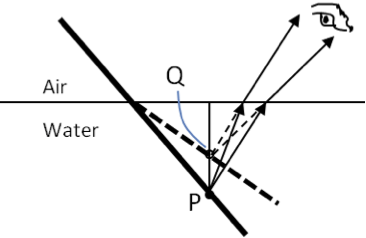Figure 47.4.1. Bending of rod at air/water interface. The point P on the rod would appear at the point Q, the image of the point P due to refraction at the interface.

To study image formation as a result of refraction, we ask the following questions:

1. What happens to the rays of light when they enter or pass through another medium?
2. Do the refracted rays originating from a single point meet at some point or diverge away from one another?

To be concrete, let us consider a simple system consisting of two media separated by a plane interface as shown in Figure 47.4.2. The object will be in one medium and the observer in the other. For instance, when you look at a fish from above the water surface, the fish would be in water which has a refractive index $\frac{4}{3}$ and your eyes woulb be in air with refractive index 1.00, and the surface of water is the interface. The depth that you will “see”, i.e., $h_i\text{,}$ will be called the apparent depth, and the actual depth of the fish will be denoted by $h_o\text{.}$

The apparent depth $h_i$ depends on the angle at which you view the image. For a view from directly above, the so-called normal view, we can assume the refraction angle $\theta$ to be small, and replace $\sin\theta$ in Snell's law by $\tan\theta\text{.}$ With this approximation, using the triangles $\triangle\text{OPR}$ and $\triangle\text{OQR}\text{,}$ you can show that the apparent depth is given by the following formula.

\begin{equation} \frac{h_i}{h_o} \approx \frac{n_2}{n_1}.\tag{47.4.1} \end{equation}

Thus, a fish will appear approximatel $\frac{3}{4}$ times the real depth when viewed from above.

Spherical shape is important in optics, primarily because high quality spherical shapes are far easier to manufacture than other specialized curved surfaces. A spherical shape can be convex if the material of higher refractive index than the surroundings is bulging out or concave if the material has been carved out. In the following subsections, we will study image formation by refraction at convex and concave surfaces. In the next section, we will study a lens made where it has two refraction surfaces.

### Subsection47.4.1Refraction at a Convex Surface

For concreteness, consider a point source of light at point P in front of a transparent material that has a convex surface facing the light source as shown in Figure 47.4.3. Let $n_1$ be the refractive index of the medium that point P is located and $n_2$ be the refractive index of the material with the convex surface. We assume that $n_2 \gt n1\text{;}$ for instance, the material may be glass with $n_2=1.5$ and the surroundings may be air with $n_1 = 1.0\text{.}$

We further assume that other surfaces of the $n_2$-material are far away and we are not interested in what happens when light ray reaches those surfaces. We want to know what happens as a result of refraction on this face only.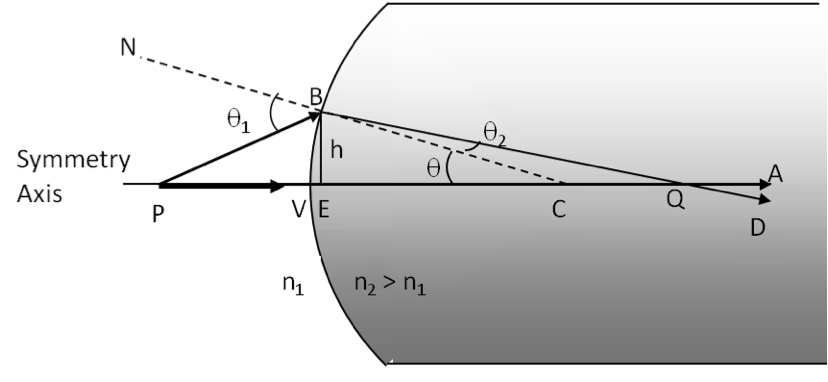Figure 47.4.3. Refraction at a convex interface with $n_2 \gt n_1\text{.}$

We will do our calculations in the paraxial approximation, i.e., for rays that make small angles with the symmetry axis. Paraxial rays make small angle $\theta\text{,}$ therefore we make the approximations $\cos\theta\approx 1$ and $\sin\theta\approx\tan\theta\approx\theta$ where $\theta$ is in radian. We also find that

\begin{align*} \amp \text{EV} \approx 0,\\ \amp n_1 \theta_ 1 \approx n_2 \theta_ 2. \end{align*}

The following geometric relations are also easily seen from the figure.

\begin{align*} \amp \text{In } \triangle \text{PBE},\ \ \ \angle \text{BPE} = \theta_1 - \theta \approx \frac{ \text{BE} }{ \text{VP} } = \frac{h}{p}.\\ \amp \text{In } \triangle \text{BEC},\ \ \ \angle \text{BCE} = \theta \approx \frac{ \text{BE} }{ \text{VC} } = \frac{h}{R}.\\ \amp \text{In } \triangle \text{QBE},\ \ \ \angle \text{BPE} = \theta - \theta_2 \approx \frac{ \text{BE} }{ \text{VQ} } = \frac{h}{q}. \end{align*}

These relations yield the following relation.

\begin{equation} \frac{n_1}{p} + \frac{n_2}{q} = \frac{n_2 - n_1}{R}. \label{eq-refraction-spherical-1}\tag{47.4.2} \end{equation}

#### Subsubsection47.4.1.1First and Second Focal Points

Figure 47.4.4 shows that there exists a special point $\text{F}_1$ in medium $n_1$ such that if you place a point object there, light passing through the convex surface becomes parallel in medium $n_2\text{.}$ This means the image of a such a source is at infinity, i.e., $q=\infty\text{.}$ Point $\text{F}_1$ is called first focal point.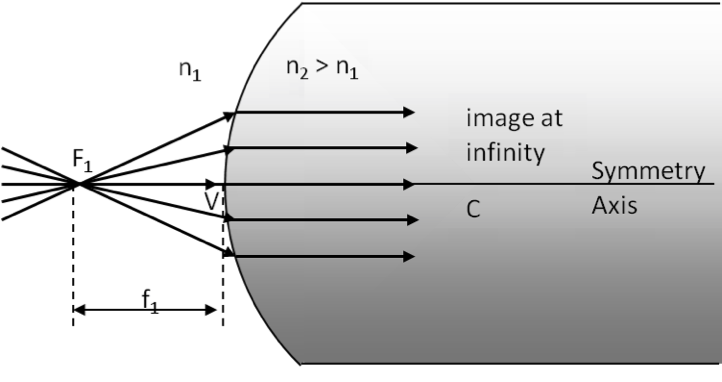Figure 47.4.4. First focal point $\text{F}_1$ for a convex spherical surface with $n_2 \gt n_1\text{.}$

With $q=\infty\text{,}$ $p$ in Eq. (47.4.2) will be equal to first focal length $f_1\text{,}$ which is the distance from vertex V to the first focal point $\text{F}_1\text{.}$

\begin{equation*} \frac{n_1}{f_1} + \frac{n_2}{\infty} = \frac{n_2 - n_1}{R}. \end{equation*}

Therefore,

\begin{equation} f_1 = \frac{n_1}{n_2 - n_1}\, R.\tag{47.4.3} \end{equation}

Figure 47.4.5 shows that there exists another special point $\text{F}_2$ in medium $n_2$ such that if you place the object very far away such that the rays from the object striking the convex surface can be considered to be parallel, e.g., source can be sunlight from the Sun. Then, we find that these rays, pass through point $\text{F}_2$ and continue. This means the image of a such a source at infinity $p=\infty\text{,}$ is at the second focal point, i.e., $q=f_2\text{.}$ Point $\text{F}_2$ is called second focal point and distance of $\text{F}_2$ from the vertex is called the second focal length.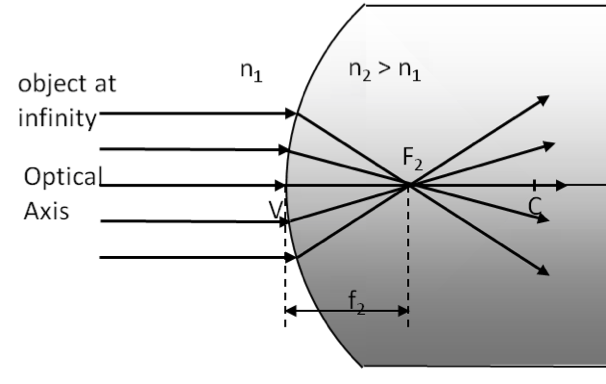Figure 47.4.5. Second focal point $\text{F}_2$ for a convex spherical surface with $n_2 \gt n_1\text{.}$

With $p=\infty\text{,}$ $q=f_2$ in Eq. (47.4.2) gives

\begin{equation*} \frac{n_1}{\infty} + \frac{n_2}{f_2} = \frac{n_2 - n_1}{R}. \end{equation*}

Therefore,

\begin{equation} f_2 = \frac{n_2}{n_2 - n_1}\, R.\tag{47.4.4} \end{equation}

### Subsection47.4.2Refraction at a Concave Surface

Consider a point source P in front of a concave surface of the medium $n_2 \gt n_1$ as shown in Figure 47.4.6. You may think of oint P being in air and the concave surface of glass if you like.

Unlike the case of refraction of rays at the convex surface, here, refracted rays, say, BD and VA diverge away from each other. Therefore they will not cross at any real image point. to find the image we extend refracted rays VA and BD backwards form then to meet at a point Q which is the virtual image of point P. Once again, we measure all distances from the vertex V. Thus VP is the object distance $p\text{,}$ VQ the image distance $q$ and VC the radius of curvature $R\text{.}$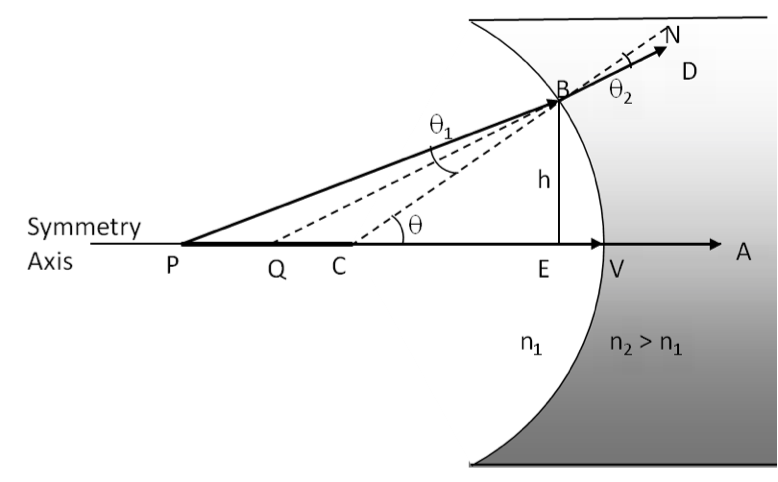Figure 47.4.6. Refraction at a concave surface with $n_2 \gt n_1\text{.}$

Using the geometry given in Figure 47.4.6, and the paraxial approximation with $\text{EV} \approx 0\text{,}$ one can derive the following relation. The derivation is left as an exercise for the student to complete.

\begin{equation*} \frac{n_1}{p} + \frac{n_2}{-q} = \frac{n_2 - n_1}{-R}. \end{equation*}

This equation for a concave surface is similar in form to Eq. (47.4.2) of the convex surface. The only differences are the negative signs with $q$ and $R\text{.}$ If we assume the following sign convention then we can write the same equation for both cases.

\begin{equation*} \frac{n_1}{p} + \frac{n_2}{q} = \frac{n_2 - n_1}{R}\ \ \text{(Sign Convention Required!)} \end{equation*}

Sign convention for refraction at spherical surfaces. Assume object is on the left of the curved surface.

1. $p$ positive.
2. $q$ positive if image to the right of the surface and negative when image to the left of the surface.
3. $R$ positive if center of circle falls on the right and negative if center on the left.

### Subsection47.4.3Transverse Magnification of Image by Refraction at Spherical Surface

I will not derive the result here, but if you draw rays from a point that is not on the axis, you can also study the transverse magnification as we did for reflection from spherical surfaces. We get similar results here.

Let $h_o$ be the height of the object, $h_i$ be the height of the image, which can be positive or negative, $p$ the object distance, and $q$ the image distance, then transverse magnification $m_T$ has the following relations.

\begin{equation} m_T = \frac{h_i}{h_o} = - \frac{q}{p}.\tag{47.4.5} \end{equation}

### Subsection47.4.4Apparent Depth Behind Curved Surface

Eq. (47.4.2) can also be applied to $n_2 \lt n_1\text{,}$ e.g., an object in a medium with higher refractive index. For instance, we had the case of fish in water. The radius of curvature in that case is infinity, $R=\infty$ since the surface is planar. This will give the following for the apparent depth, which is the value of $q$ in (47.4.2).

\begin{equation*} \frac{n_1}{p} + \frac{n_2}{q} = 0. \end{equation*}

In the fish in water tank example, we have $h_o = p$ and $h_i=-q$ since the image is on the same side as the object. The refractive indices there are $n_1=\frac{4}{3}$ and $n_2=1.0\text{.}$

\begin{equation*} \frac{h_i}{h_o} = \frac{n_2}{n_1} = \frac{3}{4}. \end{equation*}

What will hapen if the surface was not plane. Suppose, you have dust particle stuck in glass with spherical surface. Suppose, the radius of curvature is $5\text{ cm}\text{,}$ and the dust particle is at a distance $p=4\text{ cm}$ from the vertex. The following calculation will give us the distance from the vertex the dust will appear from air. Let $n_1=1.5$ for glass and $n_2=1.0$ for glass.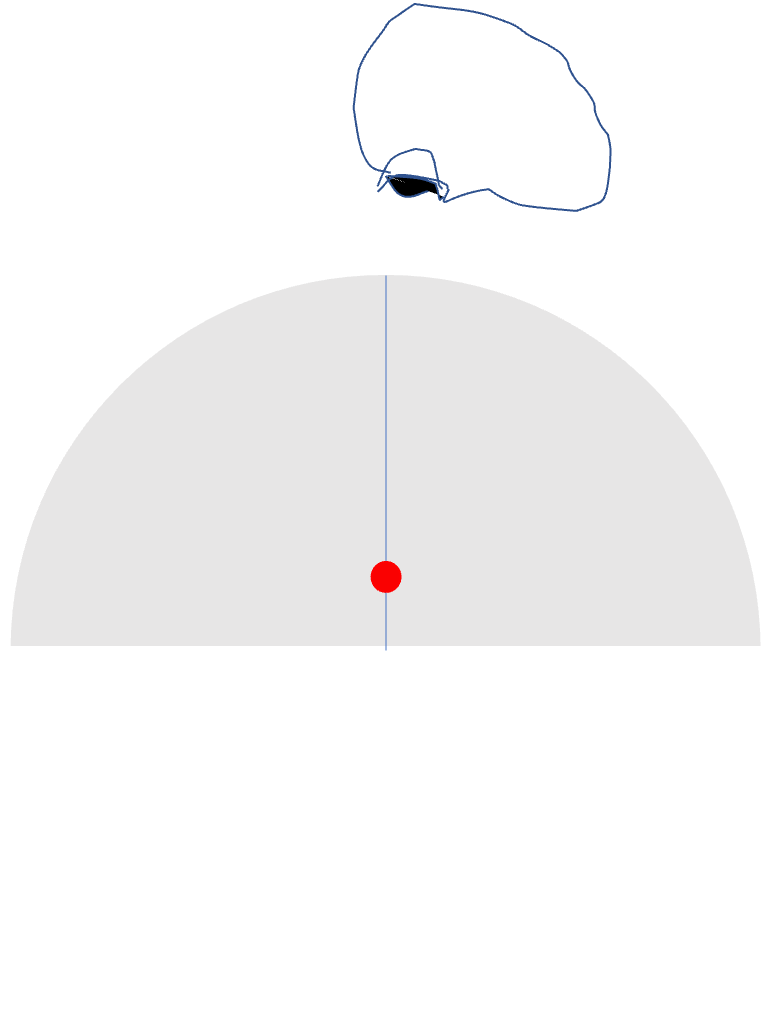\begin{equation*} \frac{1.5}{4\text{ cm}} + \frac{1.0}{q} = \frac{1.0-1.5}{5\text{ cm}}. \end{equation*}

Solving for $q$ we get

\begin{equation*} q = -\frac{40}{19}\approx -2.0\text{ cm}. \end{equation*}

The dust will be at a distance $2\text{ cm}$ from the vertex - still on the glass side since it is negative, meaning same side as the object.

An object is located in air 30 cm from the vertex of a concave surface made of glass with a radius of curvature 10 cm. Where does the image by refraction form and what are its orientation and magnification? Use $n_{\textrm{air}}$ = 1 and $n_{\textrm{glass}}$ = 1.5.

Hint

Use the formulas derived in this section.

$q= -18\text{ cm}\text{,;}$ $m = +3/5\text{.}$

Solution

In this problem we have

\begin{equation*} R = -10\:\text{cm},\ \ p = 30\:\text{cm},\ \ n_1 = 1,\ \ n_2 = 1.5. \end{equation*}

Therefore,

\begin{equation*} \dfrac{n_1}{p} + \dfrac{n_2}{q} = \dfrac{(n_2-n_1)}{R}\ \Longrightarrow\ \dfrac{1}{30} + \dfrac{1.5}{q} = \dfrac{(1.5-1)}{-10}. \end{equation*}

This gives $q = -18\:\textrm{cm}\text{.}$ The magnification of the image would be

\begin{equation*} m_T = \dfrac{h_i}{h_o} = -\dfrac{q}{p} = +\dfrac{3}{5}. \end{equation*}

Since $m_T \gt 0$ the image has the same orientation as the object.

An object is located in air $30\text{ cm}$ from the vertex of a convex surface made of glass with a radius of curvature $80\text{ cm}\text{.}$

(a) Where does the image by refraction form?

(b) What are magnification and orientation of the image relative to the object?

Hint

Use the formulas derived in this section.

$(a) q = - 55.4\text{ cm}\text{,}$ (b) $m_T = +1.85\text{.}$

Solution 1 (a)

(a) In this problem we have

\begin{equation*} R = +80\:\text{cm},\ \ p = 30\:\text{cm},\ \ n_1 = 1,\ \ n_2 = 1.5. \end{equation*}

Therefore,

\begin{equation*} \dfrac{n_1}{p} + \dfrac{n_2}{q} = \dfrac{(n_2-n_1)}{R}\ \Longrightarrow\ \dfrac{1}{30} + \dfrac{1.5}{q} = \dfrac{(1.5-1)}{80}. \end{equation*}

Therefore

\begin{equation*} \dfrac{1.5}{q} = \dfrac{1}{160} - \dfrac{1}{30} = \dfrac{3-16}{480} = -\dfrac{13}{480} \end{equation*}

This gives

\begin{equation*} q = - 1.5\times\dfrac{480}{13} = -55.4\:\text{cm}. \end{equation*}
Solution 2 (b)

(b) Since $q\lt 0$ the image is on the left of the vertex, meaning 550 cm in the air. The magnification of the image would be

\begin{equation*} m_T = \dfrac{h_i}{h_o} = -\dfrac{q}{p} = +1.85. \end{equation*}

Since $m_T \gt 0$ the image has the same orientation as the object.